Скачать презентацию 1 Outline 6 Hours of Edification

• Количество слайдов: 36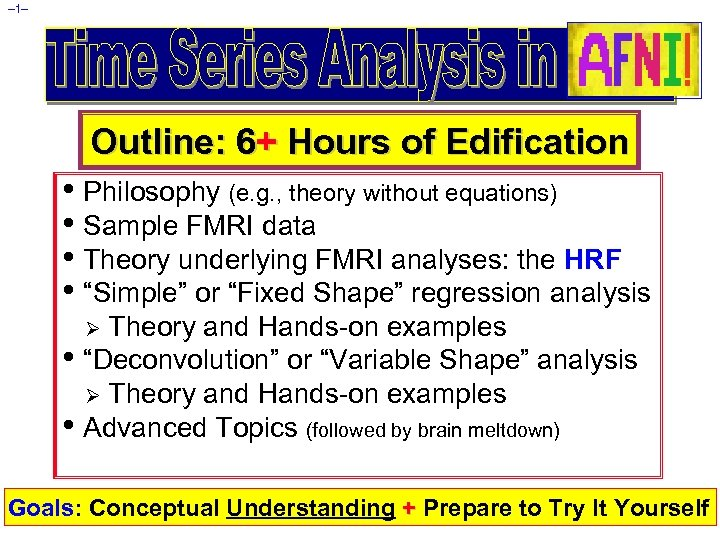– 1– Outline: 6+ Hours of Edification • Philosophy (e. g. , theory without equations) • Sample FMRI data • Theory underlying FMRI analyses: the HRF • “Simple” or “Fixed Shape” regression analysis Theory and Hands-on examples • “Deconvolution” or “Variable Shape” analysis Ø Theory and Hands-on examples • Advanced Topics (followed by brain meltdown) Ø Goals: Conceptual Understanding + Prepare to Try It Yourself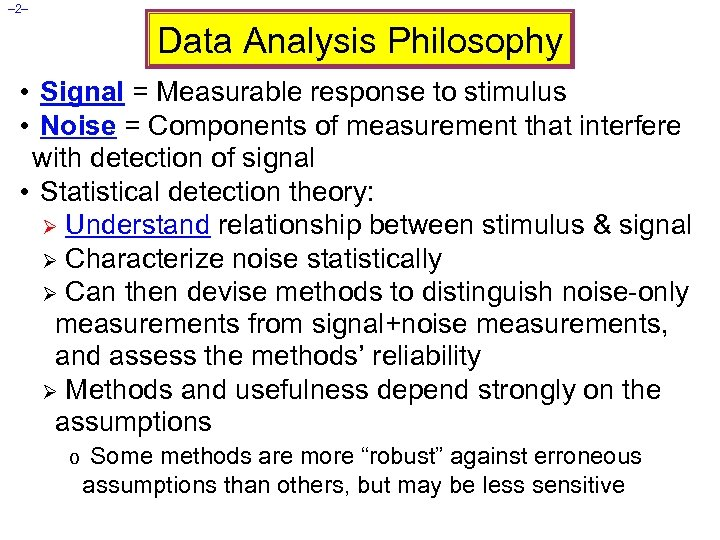– 2– Data Analysis Philosophy • Signal = Measurable response to stimulus • Noise = Components of measurement that interfere with detection of signal • Statistical detection theory: Ø Understand relationship between stimulus & signal Ø Characterize noise statistically Ø Can then devise methods to distinguish noise-only measurements from signal+noise measurements, and assess the methods’ reliability Ø Methods and usefulness depend strongly on the assumptions o Some methods are more “robust” against erroneous assumptions than others, but may be less sensitive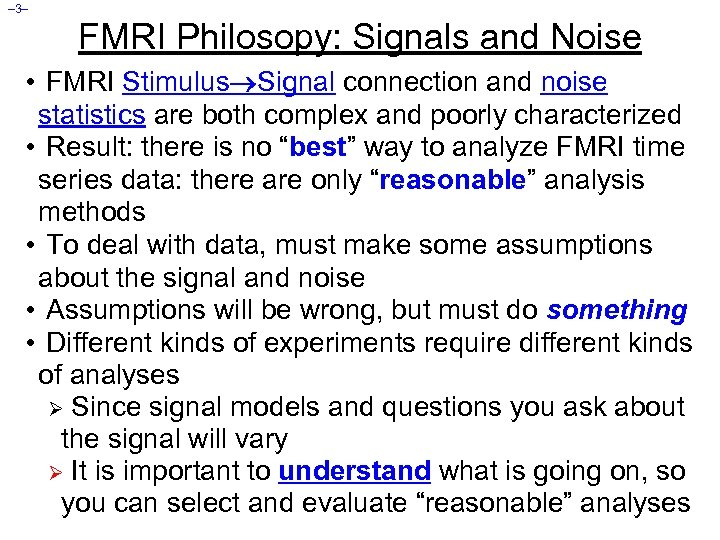– 3– FMRI Philosopy: Signals and Noise • FMRI Stimulus Signal connection and noise statistics are both complex and poorly characterized • Result: there is no “best” way to analyze FMRI time series data: there are only “reasonable” analysis methods • To deal with data, must make some assumptions about the signal and noise • Assumptions will be wrong, but must do something • Different kinds of experiments require different kinds of analyses Ø Since signal models and questions you ask about the signal will vary Ø It is important to understand what is going on, so you can select and evaluate “reasonable” analyses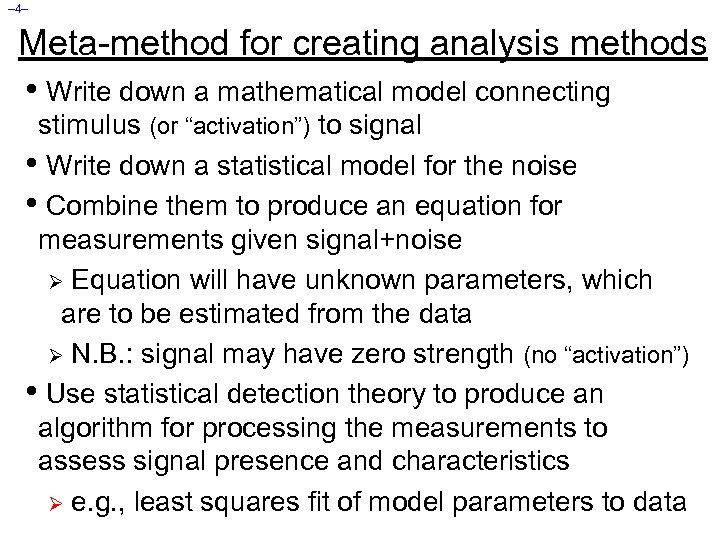– 4– Meta-method for creating analysis methods • Write down a mathematical model connecting stimulus (or “activation”) to signal • Write down a statistical model for the noise • Combine them to produce an equation for measurements given signal+noise Ø Equation will have unknown parameters, which are to be estimated from the data Ø N. B. : signal may have zero strength (no “activation”) • Use statistical detection theory to produce an algorithm for processing the measurements to assess signal presence and characteristics Ø e. g. , least squares fit of model parameters to data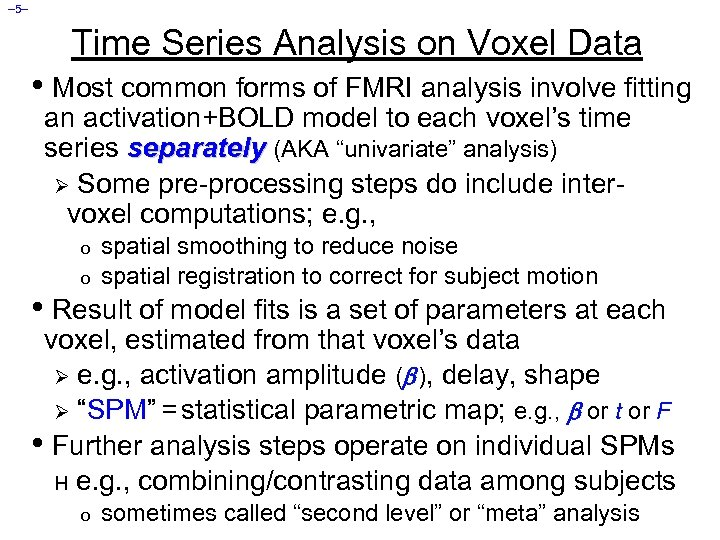– 5– Time Series Analysis on Voxel Data • Most common forms of FMRI analysis involve fitting an activation+BOLD model to each voxel’s time series separately (AKA “univariate” analysis) Ø Some pre-processing steps do include intervoxel computations; e. g. , o o spatial smoothing to reduce noise spatial registration to correct for subject motion • Result of model fits is a set of parameters at each voxel, estimated from that voxel’s data Ø e. g. , activation amplitude ( ), delay, shape Ø “SPM” = statistical parametric map; e. g. , or t or F • Further analysis steps operate on individual SPMs H e. g. , combining/contrasting data among subjects o sometimes called “second level” or “meta” analysis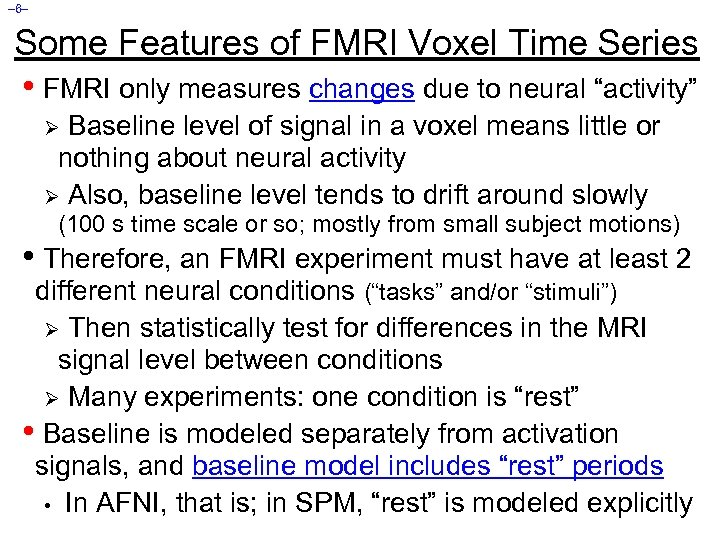– 6– Some Features of FMRI Voxel Time Series • FMRI only measures changes due to neural “activity” Baseline level of signal in a voxel means little or nothing about neural activity Ø Also, baseline level tends to drift around slowly Ø (100 s time scale or so; mostly from small subject motions) • Therefore, an FMRI experiment must have at least 2 different neural conditions (“tasks” and/or “stimuli”) Ø Then statistically test for differences in the MRI signal level between conditions Ø Many experiments: one condition is “rest” • Baseline is modeled separately from activation signals, and baseline model includes “rest” periods • In AFNI, that is; in SPM, “rest” is modeled explicitly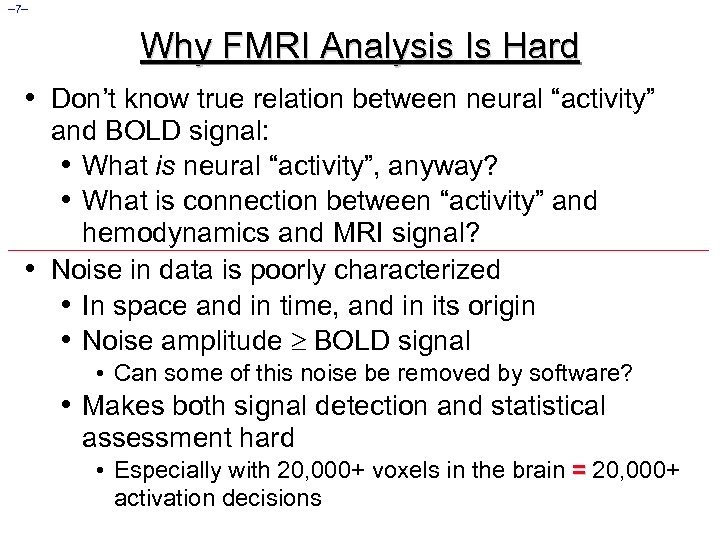– 7– Why FMRI Analysis Is Hard • Don’t know true relation between neural “activity” • and BOLD signal: • What is neural “activity”, anyway? • What is connection between “activity” and hemodynamics and MRI signal? Noise in data is poorly characterized • In space and in time, and in its origin • Noise amplitude BOLD signal • Can some of this noise be removed by software? • Makes both signal detection and statistical assessment hard • Especially with 20, 000+ voxels in the brain = 20, 000+ activation decisions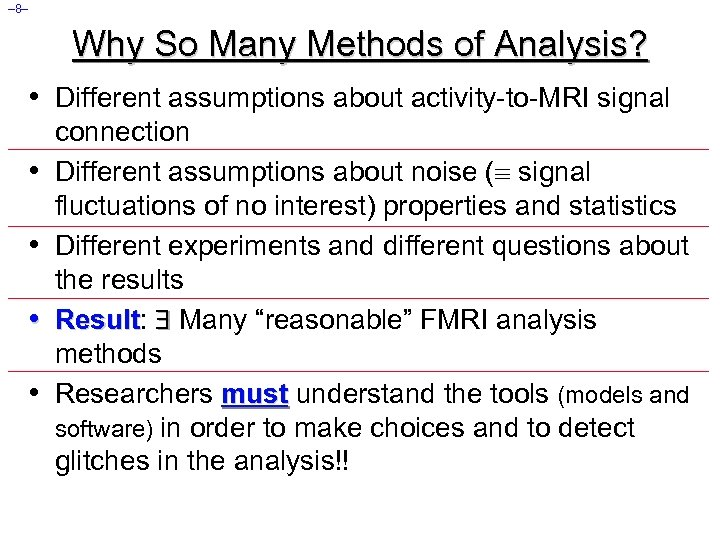– 8– Why So Many Methods of Analysis? • Different assumptions about activity-to-MRI signal • • connection Different assumptions about noise ( signal fluctuations of no interest) properties and statistics Different experiments and different questions about the results Result: Many “reasonable” FMRI analysis Result methods Researchers must understand the tools (models and software) in order to make choices and to detect glitches in the analysis!!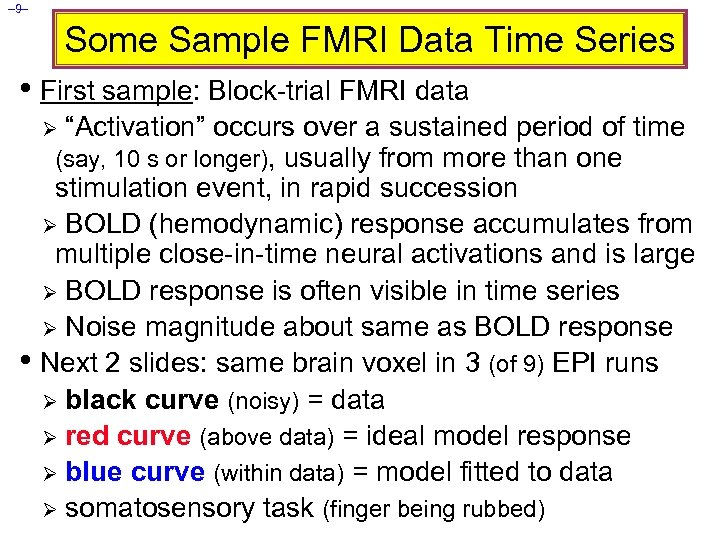– 9– Some Sample FMRI Data Time Series • First sample: Block-trial FMRI data “Activation” occurs over a sustained period of time (say, 10 s or longer), usually from more than one stimulation event, in rapid succession Ø BOLD (hemodynamic) response accumulates from multiple close-in-time neural activations and is large Ø BOLD response is often visible in time series Ø Noise magnitude about same as BOLD response • Next 2 slides: same brain voxel in 3 (of 9) EPI runs Ø black curve (noisy) = data Ø red curve (above data) = ideal model response Ø blue curve (within data) = model fitted to data Ø somatosensory task (finger being rubbed) Ø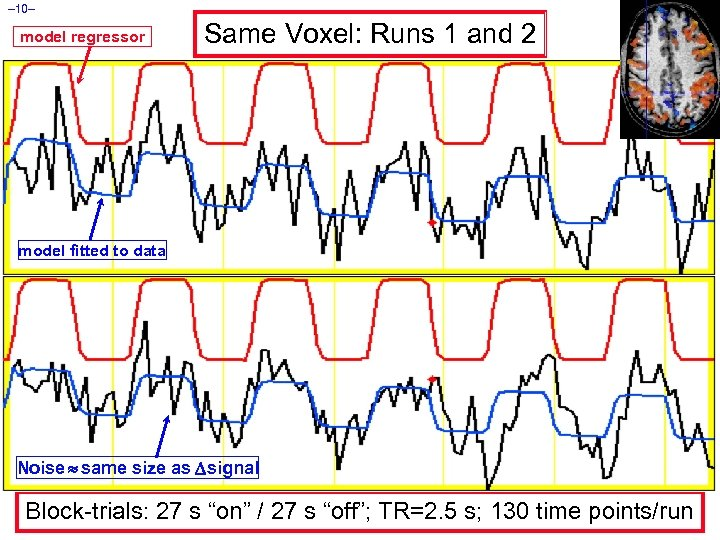– 10– model regressor Same Voxel: Runs 1 and 2 model fitted to data Noise same size as signal Block-trials: 27 s “on” / 27 s “off”; TR=2. 5 s; 130 time points/run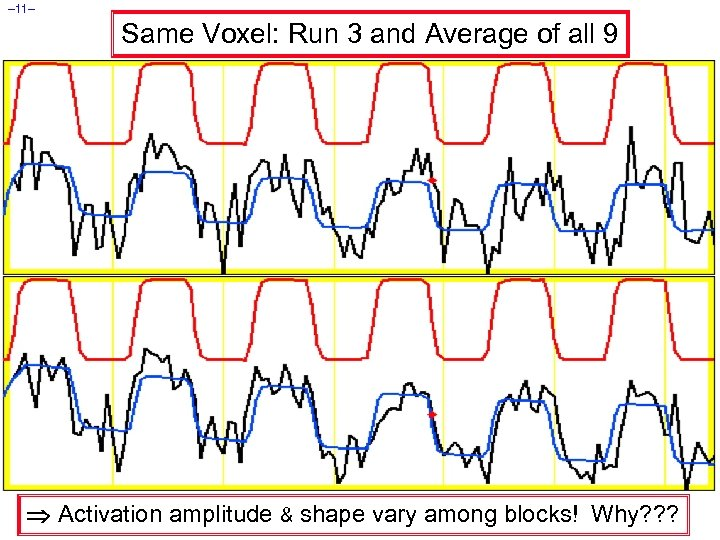– 11– Same Voxel: Run 3 and Average of all 9 Activation amplitude & shape vary among blocks! Why? ? ?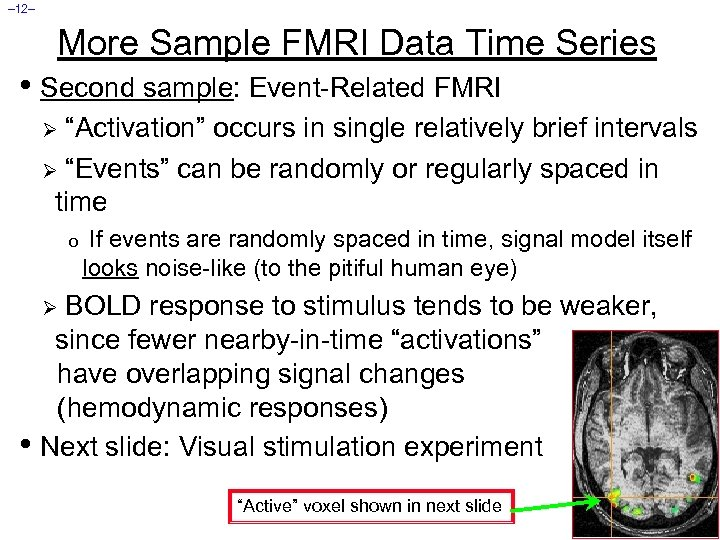– 12– More Sample FMRI Data Time Series • Second sample: Event-Related FMRI “Activation” occurs in single relatively brief intervals Ø “Events” can be randomly or regularly spaced in time Ø o If events are randomly spaced in time, signal model itself looks noise-like (to the pitiful human eye) BOLD response to stimulus tends to be weaker, since fewer nearby-in-time “activations” have overlapping signal changes (hemodynamic responses) • Next slide: Visual stimulation experiment Ø “Active” voxel shown in next slide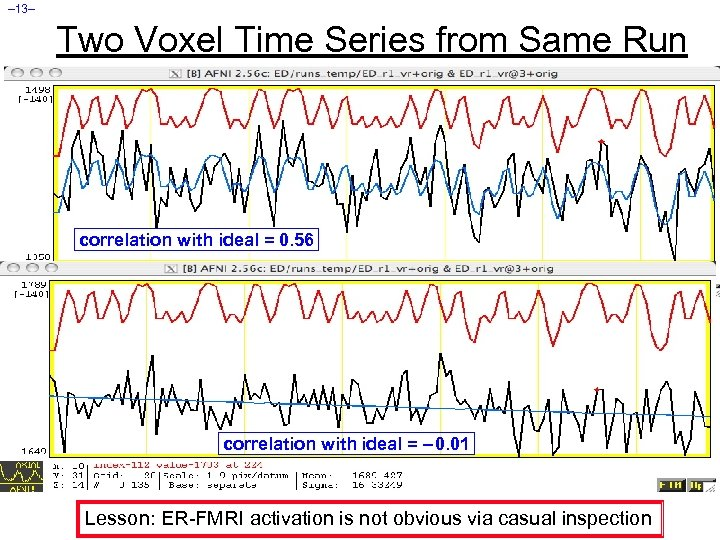– 13– Two Voxel Time Series from Same Run correlation with ideal = 0. 56 correlation with ideal = – 0. 01 Lesson: ER-FMRI activation is not obvious via casual inspection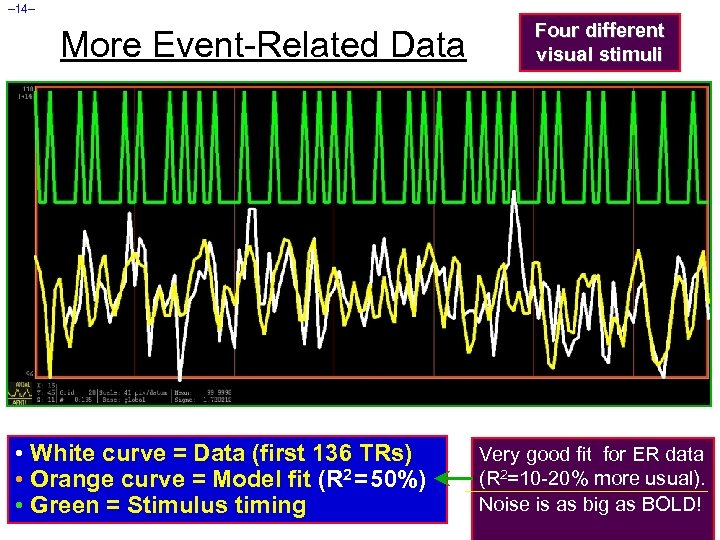– 14– More Event-Related Data • White curve = Data (first 136 TRs) • Orange curve = Model fit (R 2 = 50%) • Green = Stimulus timing Four different visual stimuli Very good fit for ER data (R 2=10 -20% more usual). Noise is as big as BOLD!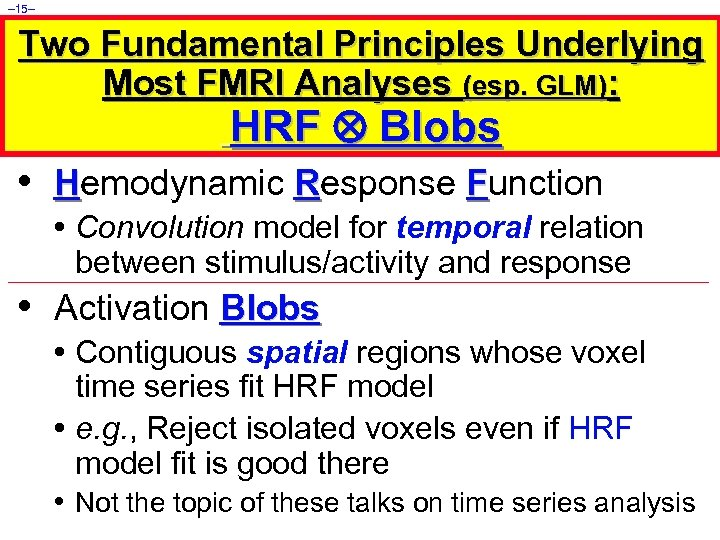– 15– Two Fundamental Principles Underlying Most FMRI Analyses (esp. GLM): HRF Blobs • Hemodynamic Response Function • Convolution model for temporal relation between stimulus/activity and response • Activation Blobs • Contiguous spatial regions whose voxel time series fit HRF model • e. g. , Reject isolated voxels even if HRF model fit is good there • Not the topic of these talks on time series analysis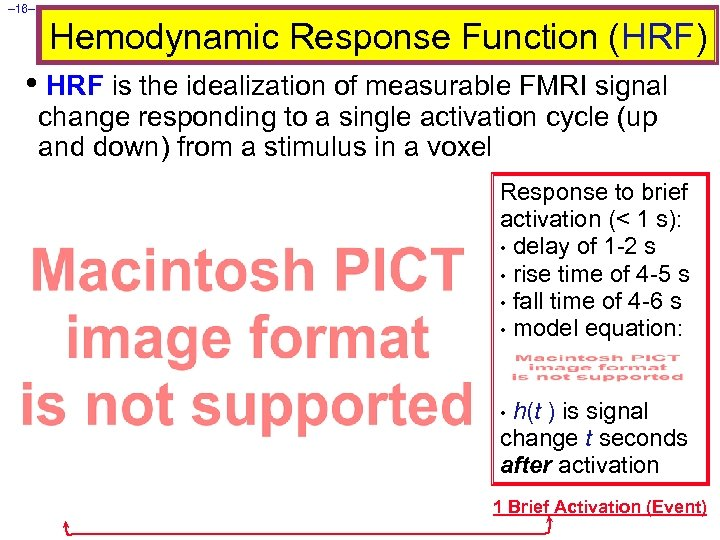– 16– Hemodynamic Response Function (HRF) • HRF is the idealization of measurable FMRI signal change responding to a single activation cycle (up and down) from a stimulus in a voxel Response to brief activation (< 1 s): • delay of 1 -2 s • rise time of 4 -5 s • fall time of 4 -6 s • model equation: h(t ) is signal change t seconds after activation • 1 Brief Activation (Event)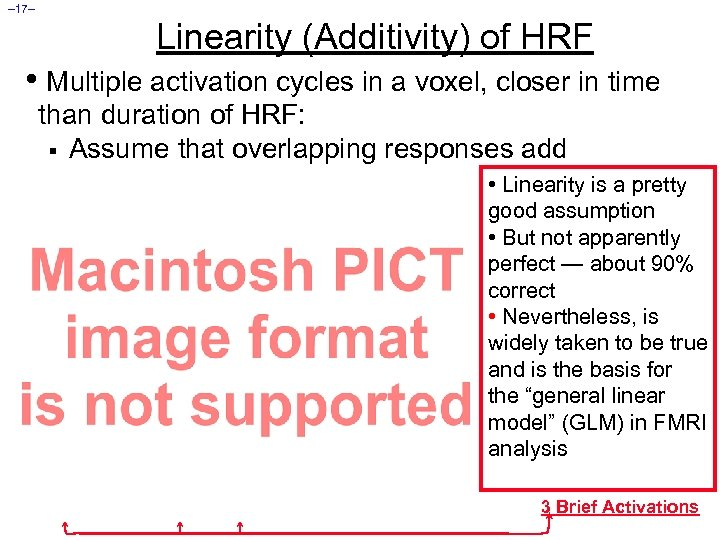– 17– Linearity (Additivity) of HRF • Multiple activation cycles in a voxel, closer in time than duration of HRF: § Assume that overlapping responses add • Linearity is a pretty good assumption • But not apparently perfect — about 90% correct • Nevertheless, is widely taken to be true and is the basis for the “general linear model” (GLM) in FMRI analysis 3 Brief Activations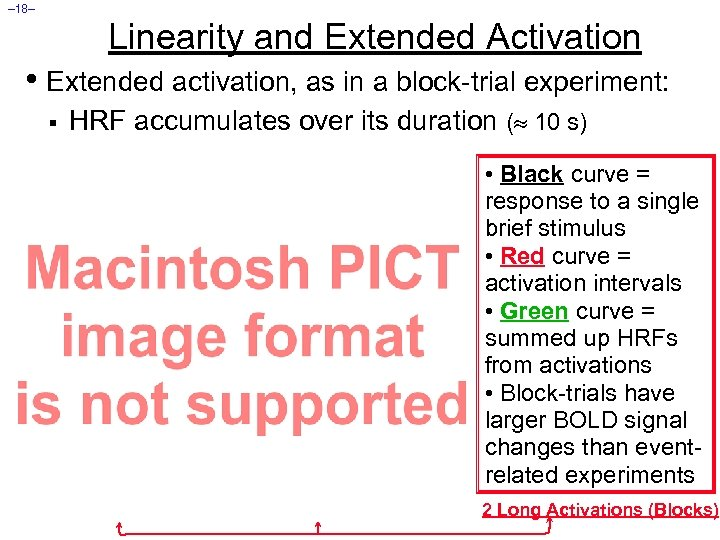– 18– Linearity and Extended Activation • Extended activation, as in a block-trial experiment: § HRF accumulates over its duration ( 10 s) • Black curve = response to a single brief stimulus • Red curve = activation intervals • Green curve = summed up HRFs from activations • Block-trials have larger BOLD signal changes than eventrelated experiments 2 Long Activations (Blocks)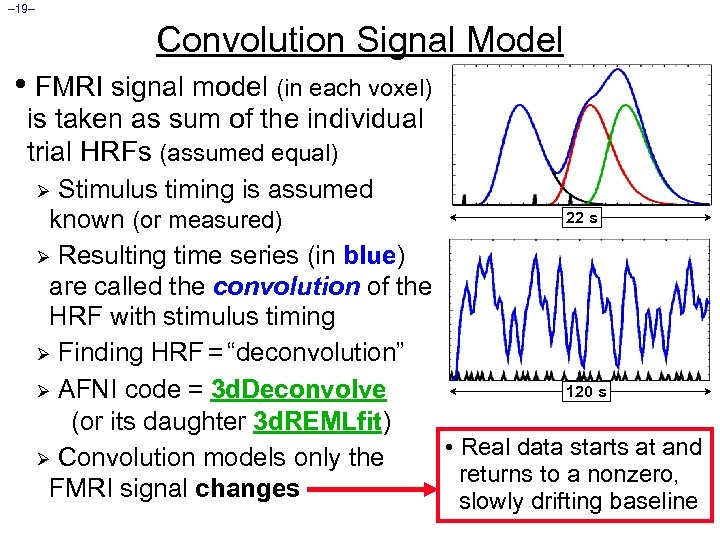– 19– Convolution Signal Model • FMRI signal model (in each voxel) is taken as sum of the individual trial HRFs (assumed equal) Stimulus timing is assumed 22 s known (or measured) Ø Resulting time series (in blue) are called the convolution of the HRF with stimulus timing Ø Finding HRF = “deconvolution” 120 s Ø AFNI code = 3 d. Deconvolve (or its daughter 3 d. REMLfit) • Real data starts at and Ø Convolution models only the returns to a nonzero, FMRI signal changes slowly drifting baseline Ø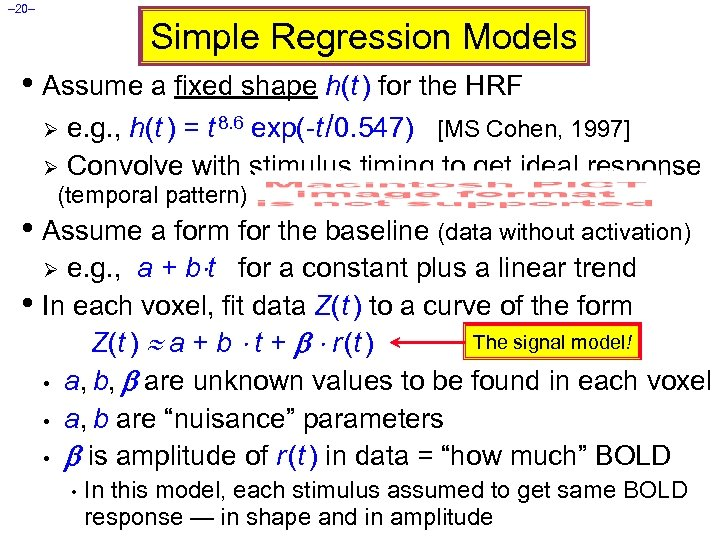– 20– Simple Regression Models • Assume a fixed shape h(t ) for the HRF e. g. , h(t ) = t 8. 6 exp(-t /0. 547) [MS Cohen, 1997] Ø Convolve with stimulus timing to get ideal response Ø (temporal pattern) • Assume a form for the baseline (data without activation) e. g. , a + b t for a constant plus a linear trend • In each voxel, fit data Z(t ) to a curve of the form The signal model! Z(t ) a + b t + r (t ) • a, b, are unknown values to be found in each voxel • a, b are “nuisance” parameters • is amplitude of r (t ) in data = “how much” BOLD Ø • In this model, each stimulus assumed to get same BOLD response — in shape and in amplitude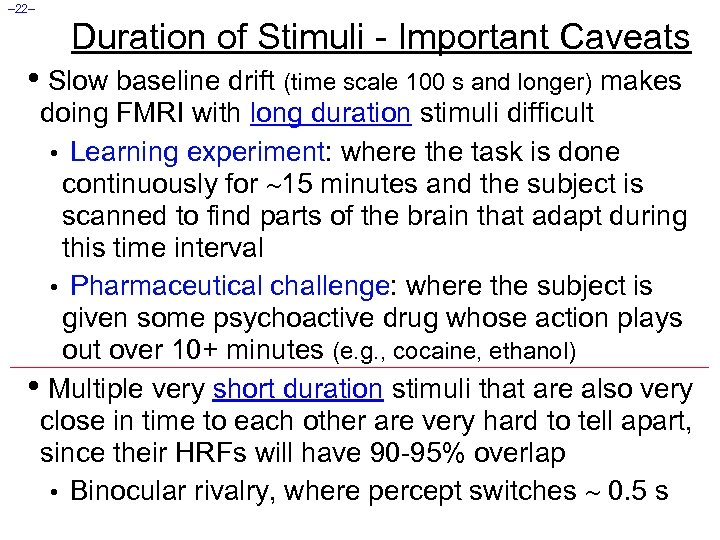– 22– Duration of Stimuli - Important Caveats • Slow baseline drift (time scale 100 s and longer) makes doing FMRI with long duration stimuli difficult • Learning experiment: where the task is done continuously for 15 minutes and the subject is scanned to find parts of the brain that adapt during this time interval • Pharmaceutical challenge: where the subject is given some psychoactive drug whose action plays out over 10+ minutes (e. g. , cocaine, ethanol) • Multiple very short duration stimuli that are also very close in time to each other are very hard to tell apart, since their HRFs will have 90 -95% overlap • Binocular rivalry, where percept switches 0. 5 s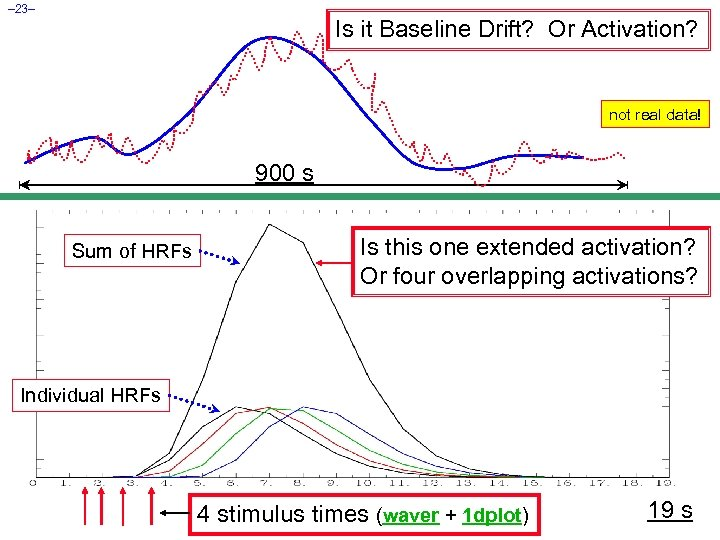– 23– Is it Baseline Drift? Or Activation? not real data! 900 s Sum of HRFs Is this one extended activation? Or four overlapping activations? Individual HRFs 4 stimulus times (waver + 1 dplot) 19 s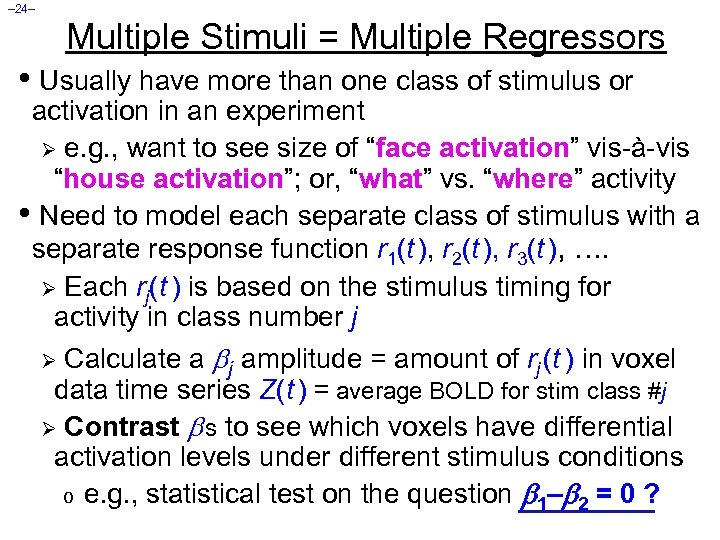– 24– Multiple Stimuli = Multiple Regressors • Usually have more than one class of stimulus or activation in an experiment Ø e. g. , want to see size of “face activation” vis-à-vis “house activation”; or, “what” vs. “where” activity • Need to model each separate class of stimulus with a separate response function r 1(t ), r 2(t ), r 3(t ), …. Ø Each rj(t ) is based on the stimulus timing for activity in class number j Ø Calculate a j amplitude = amount of rj (t ) in voxel data time series Z(t ) = average BOLD for stim class #j Ø Contrast s to see which voxels have differential activation levels under different stimulus conditions o e. g. , statistical test on the question 1– 2 = 0 ?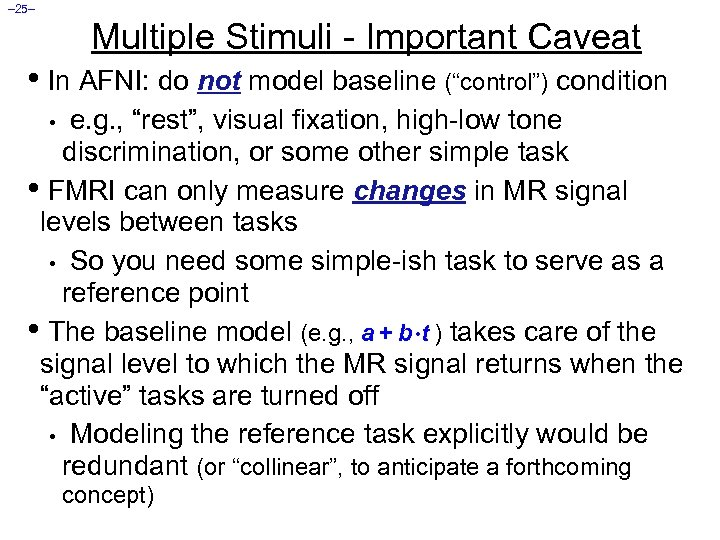– 25– Multiple Stimuli - Important Caveat • In AFNI: do not model baseline (“control”) condition e. g. , “rest”, visual fixation, high-low tone discrimination, or some other simple task • FMRI can only measure changes in MR signal levels between tasks • So you need some simple-ish task to serve as a reference point • The baseline model (e. g. , a + b t ) takes care of the signal level to which the MR signal returns when the “active” tasks are turned off • Modeling the reference task explicitly would be redundant (or “collinear”, to anticipate a forthcoming • concept)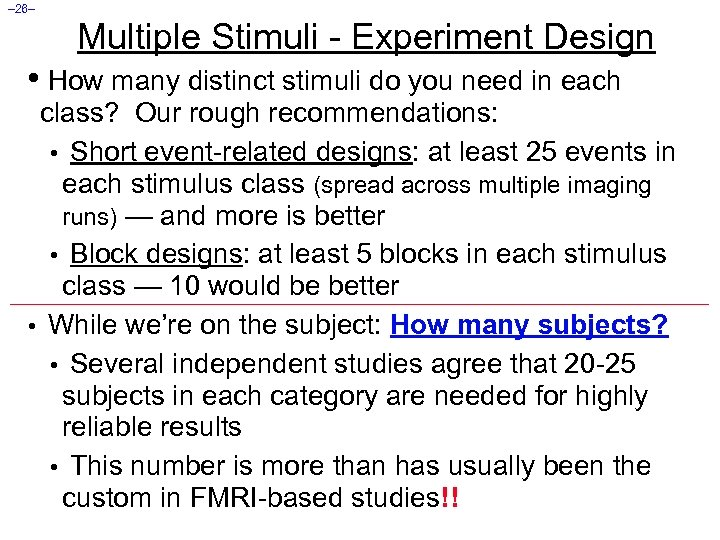– 26– Multiple Stimuli - Experiment Design • How many distinct stimuli do you need in each class? Our rough recommendations: • Short event-related designs: at least 25 events in each stimulus class (spread across multiple imaging runs) — and more is better • Block designs: at least 5 blocks in each stimulus class — 10 would be better • While we’re on the subject: How many subjects? • Several independent studies agree that 20 -25 subjects in each category are needed for highly reliable results • This number is more than has usually been the custom in FMRI-based studies!!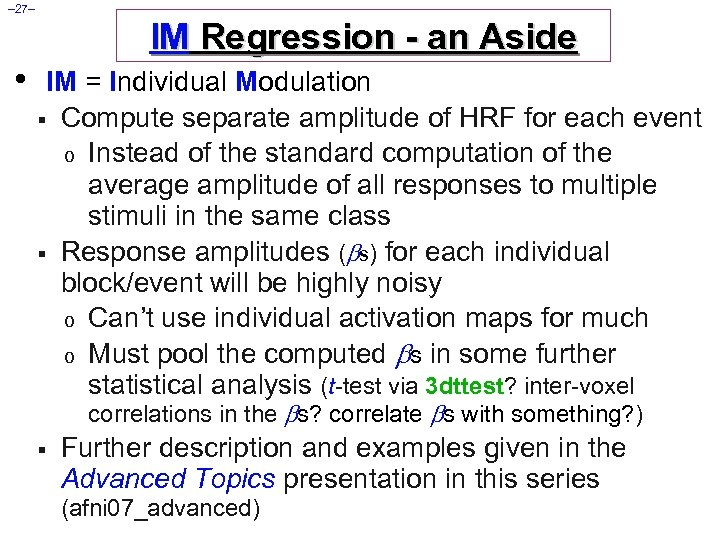– 27– IM Regression - an Aside • IM = Individual Modulation § Compute separate amplitude of HRF for each event o Instead of the standard computation of the average amplitude of all responses to multiple stimuli in the same class § Response amplitudes ( s) for each individual block/event will be highly noisy o Can’t use individual activation maps for much o Must pool the computed s in some further statistical analysis (t-test via 3 dttest? inter-voxel correlations in the s? correlate s with something? ) § Further description and examples given in the Advanced Topics presentation in this series (afni 07_advanced)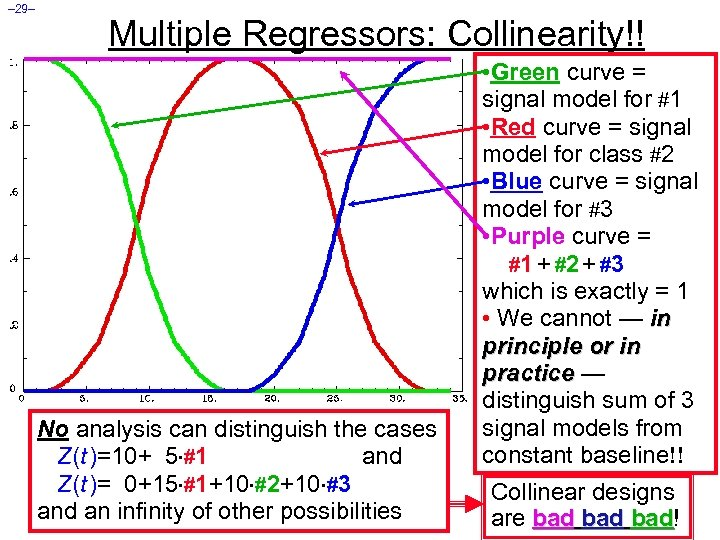– 29– Multiple Regressors: Collinearity!! No analysis can distinguish the cases Z(t )=10+ 5 #1 and Z(t )= 0+15 #1+10 #2+10 #3 and an infinity of other possibilities • Green curve = signal model for #1 • Red curve = signal model for class #2 • Blue curve = signal model for #3 • Purple curve = #1 + #2 + #3 which is exactly = 1 • We cannot — in principle or in practice — distinguish sum of 3 signal models from constant baseline!! Collinear designs are bad bad! bad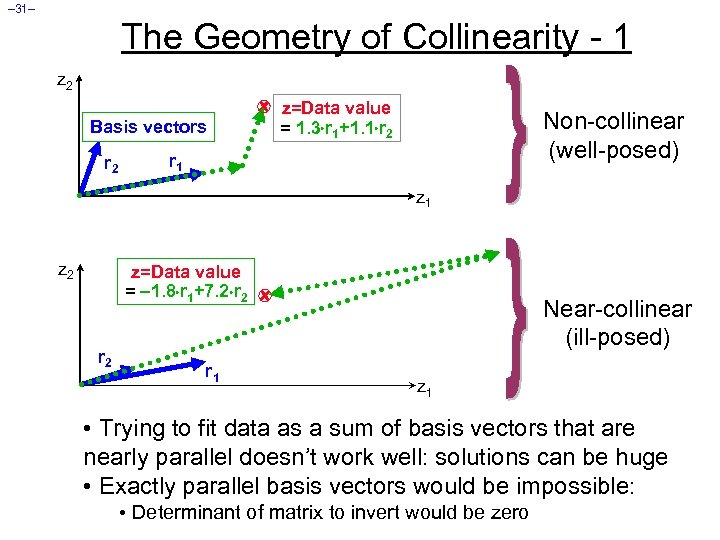– 31– The Geometry of Collinearity - 1 z 2 Basis vectors r 2 z=Data value = 1. 3 r 1+1. 1 r 2 Non-collinear (well-posed) r 1 z 2 z=Data value = 1. 8 r 1+7. 2 r 2 r 1 Near-collinear (ill-posed) z 1 • Trying to fit data as a sum of basis vectors that are nearly parallel doesn’t work well: solutions can be huge • Exactly parallel basis vectors would be impossible: • Determinant of matrix to invert would be zero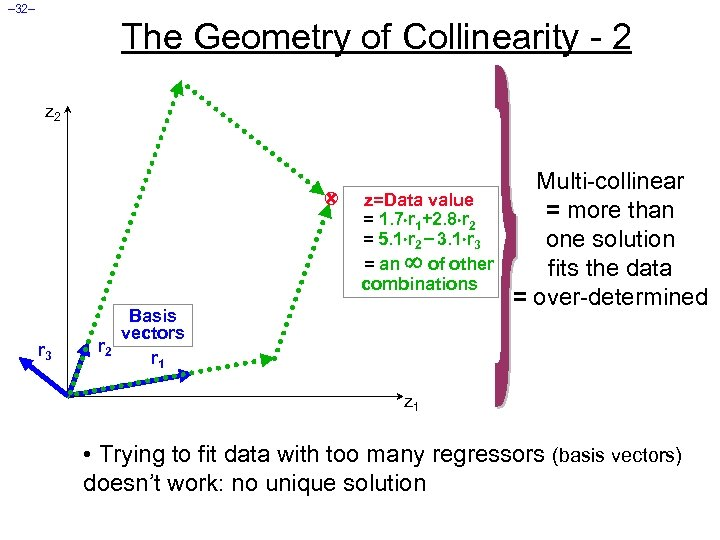– 32– The Geometry of Collinearity - 2 z=Data value = 1. 7 r 1+2. 8 r 2 = 5. 1 r 2 3. 1 r 3 = an of other combinations r 3 Basis vectors r 2 r 1 Multi-collinear = more than one solution fits the data = over-determined z 1 • Trying to fit data with too many regressors (basis vectors) doesn’t work: no unique solution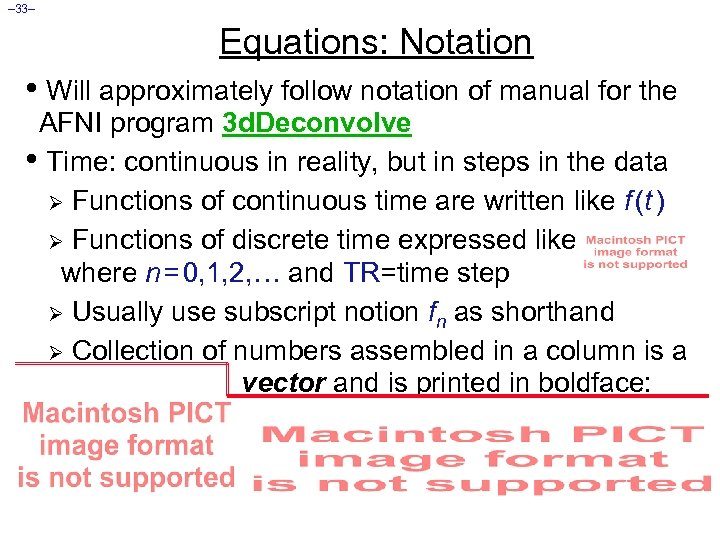– 33– Equations: Notation • Will approximately follow notation of manual for the AFNI program 3 d. Deconvolve • Time: continuous in reality, but in steps in the data Ø Functions of continuous time are written like f (t ) Ø Functions of discrete time expressed like where n = 0, 1, 2, … and TR=time step Ø Usually use subscript notion fn as shorthand Ø Collection of numbers assembled in a column is a vector and is printed in boldface: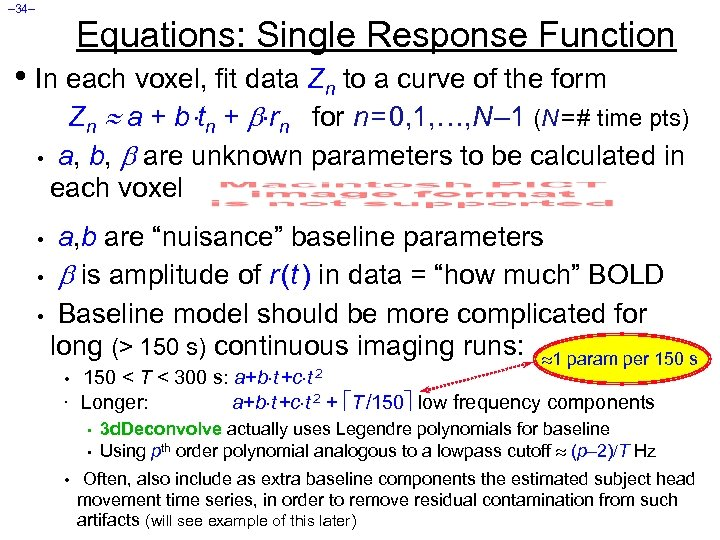– 34– Equations: Single Response Function • In each voxel, fit data Zn to a curve of the form Zn a + b tn + rn for n = 0, 1, …, N – 1 (N = # time pts) • a, b, are unknown parameters to be calculated in each voxel a, b are “nuisance” baseline parameters • is amplitude of r (t ) in data = “how much” BOLD • Baseline model should be more complicated for long (> 150 s) continuous imaging runs: 1 param per 150 s • • • 150 < T < 300 s: a+b t +c t 2 Longer: a+b t +c t 2 + T /150 low frequency components • • • 3 d. Deconvolve actually uses Legendre polynomials for baseline Using pth order polynomial analogous to a lowpass cutoff (p 2) T Hz Often, also include as extra baseline components the estimated subject head movement time series, in order to remove residual contamination from such artifacts (will see example of this later)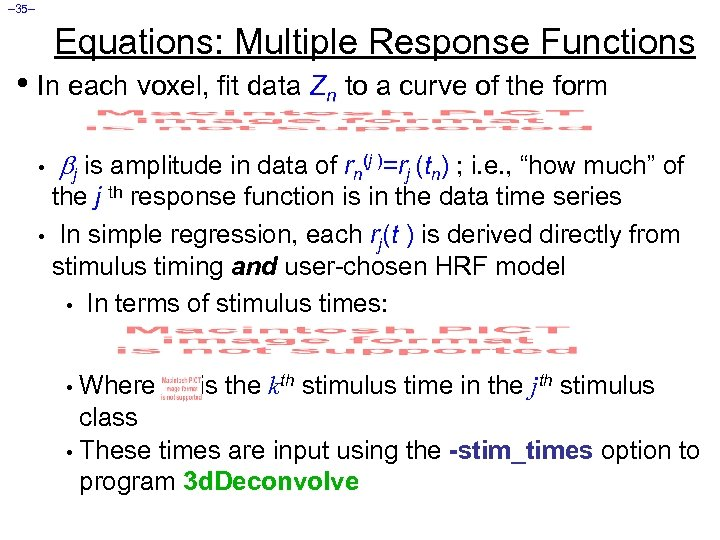– 35– Equations: Multiple Response Functions • In each voxel, fit data Zn to a curve of the form • j is amplitude in data of rn(j )=rj (tn) ; i. e. , “how much” of the j th response function is in the data time series • In simple regression, each rj(t ) is derived directly from stimulus timing and user-chosen HRF model • In terms of stimulus times: Where is the kth stimulus time in the jth stimulus class • These times are input using the -stim_times option to program 3 d. Deconvolve •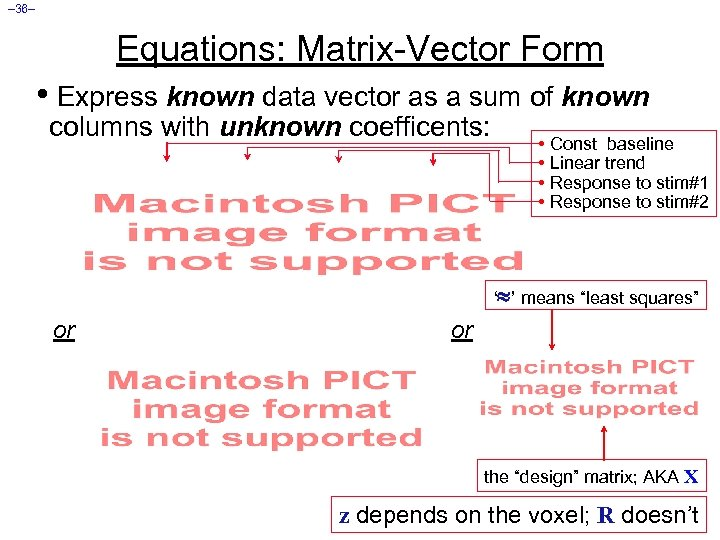– 36– Equations: Matrix-Vector Form • Express known data vector as a sum of known columns with unknown coefficents: • Const baseline • Linear trend • Response to stim#1 • Response to stim#2 ‘ ’ means “least squares” or or the “design” matrix; AKA X z depends on the voxel; R doesn’t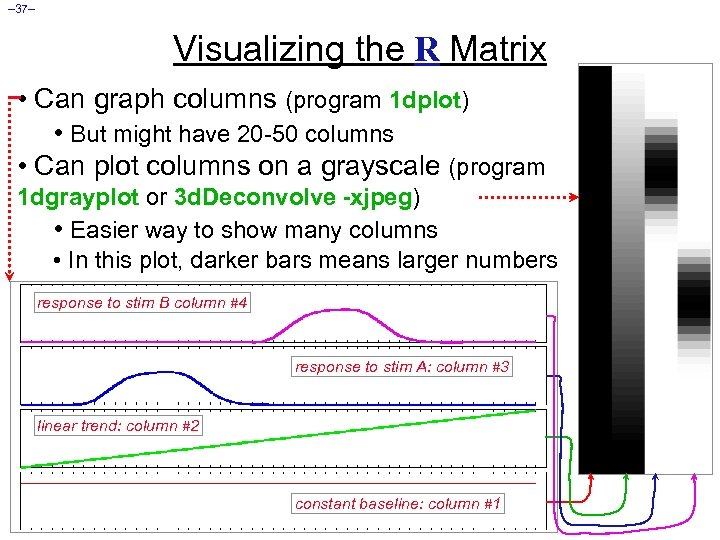– 37– Visualizing the R Matrix • Can graph columns (program 1 dplot) • But might have 20 -50 columns • Can plot columns on a grayscale (program 1 dgrayplot or 3 d. Deconvolve -xjpeg) • Easier way to show many columns • In this plot, darker bars means larger numbers response to stim B column #4 response to stim A: column #3 linear trend: column #2 constant baseline: column #1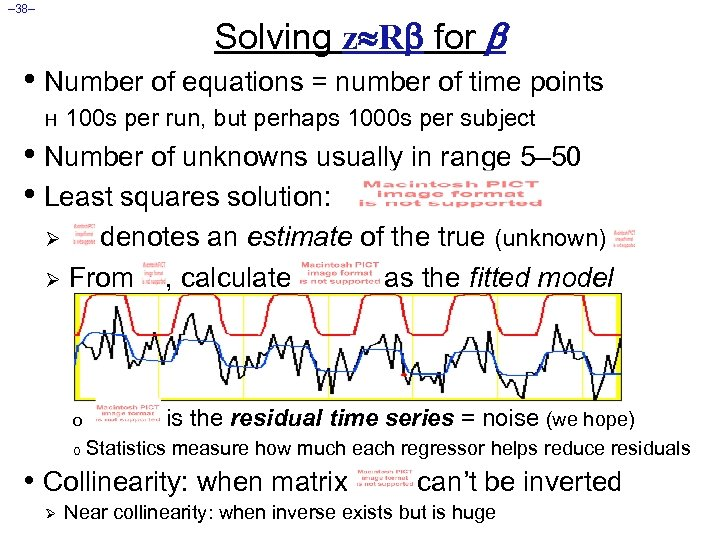– 38– Solving z R for • Number of equations = number of time points H 100 s per run, but perhaps 1000 s per subject • Number of unknowns usually in range 5– 50 • Least squares solution: denotes an estimate of the true (unknown) Ø From , calculate as the fitted model Ø o o is the residual time series = noise (we hope) Statistics measure how much each regressor helps reduce residuals • Collinearity: when matrix Ø can’t be inverted Near collinearity: when inverse exists but is huge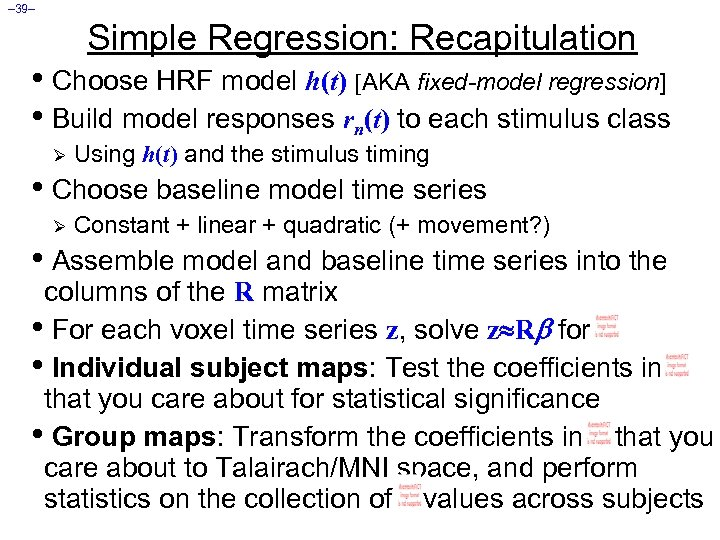– 39– Simple Regression: Recapitulation • Choose HRF model h(t) [AKA fixed-model regression] • Build model responses rn(t) to each stimulus class Ø Using h(t) and the stimulus timing • Choose baseline model time series Ø Constant + linear + quadratic (+ movement? ) • Assemble model and baseline time series into the columns of the R matrix • For each voxel time series z, solve z R for • Individual subject maps: Test the coefficients in that you care about for statistical significance • Group maps: Transform the coefficients in that you care about to Talairach/MNI space, and perform statistics on the collection of values across subjects